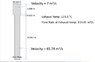# Can someone help me to calculate the velocity at Stack Tip?

What is the "right"?In summary, the question is about calculating the velocity at the stack tip with a distance of 2.5 m for the larger diameter. The attempt at a solution involved using the equation V1A1 = V2A2, but the user realizes that this equation may not apply to compressible fluids like flue gases. They also mention using the gas equation to solve the issue. In the conversation, there is also a mention of confusion in the diagram regarding the units for velocity and flow rate. f

## Homework Statement

How can we calculate the velocity at stack tip if the distance of larger diameter is 2.5 m?
I think i cannot use the equation of V1A1 = V2A2 because they may have some effect from 2.5 m of distance.
Thank you very much.

Q1=Q2

## The Attempt at a Solution

V1A1 = V2A2
45.74*2.4 = V2*6.16
V2 = 17.82 m/s

#### Attachments

•Exhaust Stack.JPG
20.6 KB · Views: 550

## Homework Statement

How can we calculate the velocity at stack tip if the distance of larger diameter is 2.5 m?
I think i cannot use the equation of V1A1 = V2A2 because they may have some effect from 2.5 m of distance.
I think you're right. That formula applies to incompressible fluids like water. The flue gases are of course compressible.

I'm afraid I must leave this one to other helpers.

•There seems to be some confusion in the diagram. It shows a "velocity" in m3/s, asks about another such in the same units, but also quotes a "flow rate" in those units at the "exhaust temperature". How does that relate to the two velocities?
I have a suspicion the two "velocities" are supposed to be m/s. Given the diameter, that would seem to be consistent with the volumetric flow rate. If so, it makes the question much easier; you can ignore most of the information.

•I think you're right. That formula applies to incompressible fluids like water. The flue gases are of course compressible.

I'm afraid I must leave this one to other helpers.
Thank you very much, now I am looking the gas equation also that can apply this issue.

There seems to be some confusion in the diagram. It shows a "velocity" in m3/s, asks about another such in the same units, but also quotes a "flow rate" in those units at the "exhaust temperature". How does that relate to the two velocities?
I have a suspicion the two "velocities" are supposed to be m/s. Given the diameter, that would seem to be consistent with the volumetric flow rate. If so, it makes the question much easier; you can ignore most of the information.
Oh!, that's my mistake, the right should be (m/s) as you said.
Thank you very much

Oh!, that's my mistake, the right should be (m/s) as you said.
Thank you very much
"The right"? Do you mean the "flow rate"? I would have thought that was m3/s, and the two "velocities" should be m/s.